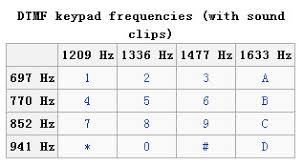## 前言## Tone(其实后来发现有错误，真实的是
222999227774442227777777733222777338866666255533355524

## Battleships

zmy大佬好像是用的正规解法，也就是存档改档读档的方法出的。回头看看他们Write up到底咋回事。

## Sender

220 ugm.cybrics.net ESMTP Postfix (Ubuntu)
EHLO localhost
250-ugm.cybrics.net
250-PIPELINING
250-SIZE 10240000
250-VRFY
250-ETRN
250-ENHANCEDSTATUSCODES
250-8BITMIME
250 DSN
334 VXNlcm5hbWU6
ZmF3a2Vz
235 2.7.0 Authentication successful
MAIL FROM: fawkes@ugm.cybrics.net
250 2.1.0 Ok
RCPT TO: area51@af.mil
250 2.1.5 Ok
DATA
354 End data with .
From: fawkes fawkes@ugm.cybrics.net
To: Area51 area51@af.mil
Content-Type: text/plain; charset="iso-8859-1"
Content-Transfer-Encoding: quoted-printable
MIME-Version: 1.0

=62=74=77=2E=0A=0A=70=61=73=73=77=6F=72=64 =66=6F=72 =74=68=65 =61=72=63=
=68=69=76=65 =77=69=74=68 =66=6C=61=67=3A =63=72=61=63=6B=30=57=65=73=74=
=6F=6E=38=38=76=65=72=74=65=62=72=61=0A=0A=63=68=65=65=72=73=21=0A
.
250 2.0.0 Ok: queued as C4D593E8B6
QUIT
221 2.0.0 Bye

btw.
password for the archive with flag: crack0Weston88vertebra
cheers!

## Paranoid

WLAN_AP_WEP_KEY1=Xi1nvy5KGSgI2&

WLAN_AP_WPA_PSK=2_RGR_xO-uiJFiAxdA33-PsdanuK&

## Telegram